# Understanding the regression line with standard units¶

This is a notebook to accompany the blog post "Understanding the regression line with standard units". Read the post for additional context!

In :
import numpy as np
from datascience import *
from datetime import *
import matplotlib
%matplotlib inline
import matplotlib.pyplot as plots


We're using the datascience package that the Data 8X course uses. Everything else we're using here is pretty standard.

To start with, let's make a table of the height and weight data we'll be working with. For now, dates are just strings, but we'll fix that later!

In :
heightweight = Table().with_columns([
'Date',        ['07/28/2017', '08/07/2017', '08/25/2017', '09/25/2017', '11/28/2017', '01/26/2018', '04/27/2018', '07/30/2018'],
'Height (cm)', [        53.3,         54.6,         55.9,           61,         63.5,         67.3,         71.1,         74.9],
'Weight (kg)', [       4.204,         4.65,        5.425,         6.41,        7.985,        9.125,        10.39,       10.785],
])
heightweight

Out:
Date Height (cm) Weight (kg)
07/28/2017 53.3 4.204
08/07/2017 54.6 4.65
08/25/2017 55.9 5.425
09/25/2017 61 6.41
11/28/2017 63.5 7.985
01/26/2018 67.3 9.125
04/27/2018 71.1 10.39
07/30/2018 74.9 10.785

Now we can plot weight as a function of height.

In :
heightweight.scatter('Height (cm)', 'Weight (kg)')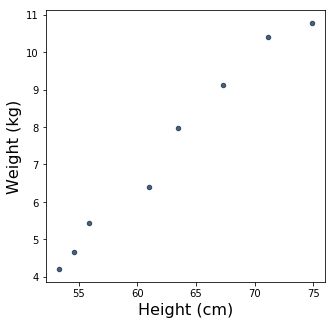Look at that! Those dots aren't too far from being a straight line. It looks as though height and weight are more or less linearly related! We'll come back to that in a moment, but first, let's convert them to standard units.

## Converting to standard units¶

A value in standard units is how many standard deviations above the mean it is. So, to convert a data point to standard units, we need the mean and the standard deviation of the data set that it came from. Then we subtract the mean from it, and then divide that by the standard deviation The standard_units function below, which comes from the Data 8 textbook, does this for a whole array of numbers at once.

In :
def standard_units(nums):
return (nums - np.mean(nums)) / np.std(nums)


Now we can create a version of the heightweight table that's in standard units.

In :
heightweight_standard = Table().with_columns(
'Date', heightweight.column('Date'),
'Height (standard units)', standard_units(heightweight.column('Height (cm)')),
'Weight (standard units)', standard_units(heightweight.column('Weight (kg)')))
heightweight_standard

Out:
Date Height (standard units) Weight (standard units)
07/28/2017 -1.26135 -1.3158
08/07/2017 -1.08691 -1.13054
08/25/2017 -0.912464 -0.808628
09/25/2017 -0.228116 -0.399485
11/28/2017 0.107349 0.254728
01/26/2018 0.617255 0.728253
04/27/2018 1.12716 1.2537
07/30/2018 1.63707 1.41777

And we can plot this data, just like we did with the data in original units.

In :
heightweight_standard.scatter('Height (standard units)', 'Weight (standard units)')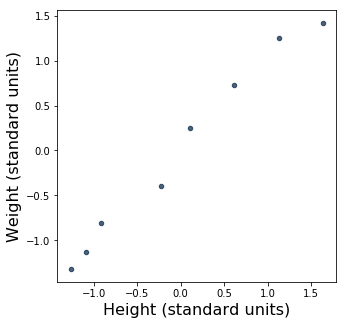The data points on our plot are exactly the same. Only the axes have changed.

## The correlation coefficient¶

Now that we've got standard units, though, it's easy to compute the correlation coefficient $r$. First, we need to take the product of our $x$ and $y$ values -- in this case, height and weight -- for each data point. We'll add a new column to our table that has these products.

In :
heightweight_product = heightweight_standard.with_column(
'Product of standardized height and weight',
heightweight_standard.column('Height (standard units)') * heightweight_standard.column('Weight (standard units)'))
heightweight_product

Out:
Date Height (standard units) Weight (standard units) Product of standardized height and weight
07/28/2017 -1.26135 -1.3158 1.65968
08/07/2017 -1.08691 -1.13054 1.22879
08/25/2017 -0.912464 -0.808628 0.737844
09/25/2017 -0.228116 -0.399485 0.091129
11/28/2017 0.107349 0.254728 0.0273447
01/26/2018 0.617255 0.728253 0.449518
04/27/2018 1.12716 1.2537 1.41312
07/30/2018 1.63707 1.41777 2.32099

To compute $r$, we just need to take the mean of the product column.

In :
r = np.mean(heightweight_product.column('Product of standardized height and weight'))
r

Out:
0.9910523777994954

Wow. $r$ is very close to 1, which means that our data has an almost perfect linear correlation.

What does "almost perfect" look like? Now that we have $r$, we can plot the regression line. (To be specific, we're plotting a segment of the regression line that goes from -2 to 2 on the $x$-axis, but we could plot a longer segment if we wanted to.)

In :
heightweight_standard.scatter('Height (standard units)', 'Weight (standard units)', label="data")
x = np.array(range(-2, 3))
y = r * x # <-- the regression equation!
plots.plot(x, y, label="regression line", linewidth=1.5)
plots.legend();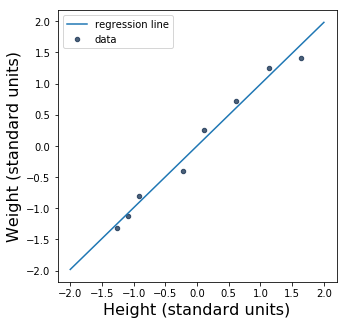Since $r$ is so close to 1, the regression line is awfully close to $y = x$, a perfect linear correlation. Let's plot that line, too.

In :
heightweight_standard.scatter('Height (standard units)', 'Weight (standard units)', label="data")
x = np.array(range(-2, 3))
y = r * x # <-- the regression equation
plots.plot(x, y, label="regression line", linewidth=1.5)
y = x # <-- linear correlation
plots.plot(x, y, label="linear correlation", linewidth=1.5)
plots.legend();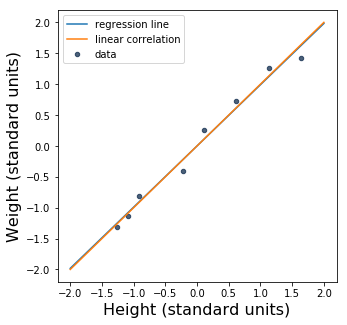The slope of the actual regression line is just sliiiiightly smaller than the slope of the line $y = x$. That is, the regression line is slightly higher than the red line on the left side of the plot, and slightly lower on the right side. If we scale up the plot size and tweak the line width and range of the axes, we can see the separation between the two lines a bit more clearly.

In :
original_dpi = plots.rcParams['figure.dpi']
plots.rcParams['figure.dpi'] = plots.rcParams['figure.dpi'] * 1.5
heightweight_standard.scatter('Height (standard units)', 'Weight (standard units)', label="data")
x = np.array(range(-2, 101))
y = r * x # <-- the regression equation
plots.plot(x, y, label="regression line", linewidth=0.5)
y = x # <-- linear correlation
plots.plot(x, y, label="linear correlation", linewidth=0.5)
plots.legend()
plots.rcParams['figure.dpi'] = original_dpi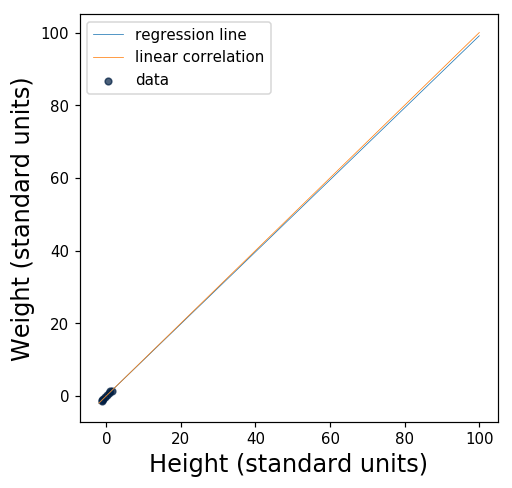## Secret Bonus Content™

We've seen that the relationship between height and weight in this data set is very well captured by a straight line. But what about the relationship between height and time, or weight and time?

Let's go back to our original table and look at the date column. To make dates easier to work with, let's convert them to Python date objects instead of strings.

In :
heightweight_dates = heightweight.with_columns(
'Date',
[datetime.strptime(date, "%m/%d/%Y").date() for date in heightweight.column(0)])
heightweight_dates

Out:
Date Height (cm) Weight (kg)
2017-07-28 53.3 4.204
2017-08-07 54.6 4.65
2017-08-25 55.9 5.425
2017-09-25 61 6.41
2017-11-28 63.5 7.985
2018-01-26 67.3 9.125
2018-04-27 71.1 10.39
2018-07-30 74.9 10.785

We can now compute the number of days since birth for each row in our data set. Sylvia was born on July 24, 2017, so we'll define that date to be her birthday and work from there.

In :
birthday = date(2017,7,24)
days_since_birth = [(date - birthday).days for date in heightweight_dates.column('Date')]
heightweight_with_days = heightweight_dates.with_columns('Days since birth', days_since_birth)
heightweight_with_days

Out:
Date Height (cm) Weight (kg) Days since birth
2017-07-28 53.3 4.204 4
2017-08-07 54.6 4.65 14
2017-08-25 55.9 5.425 32
2017-09-25 61 6.41 63
2017-11-28 63.5 7.985 127
2018-01-26 67.3 9.125 186
2018-04-27 71.1 10.39 277
2018-07-30 74.9 10.785 371

And now we can easily plot the relationship between days since birth and height, and the relationship between days since birth and weight.

In :
heightweight_with_days.scatter('Days since birth', 'Height (cm)')
heightweight_with_days.scatter('Days since birth', 'Weight (kg)')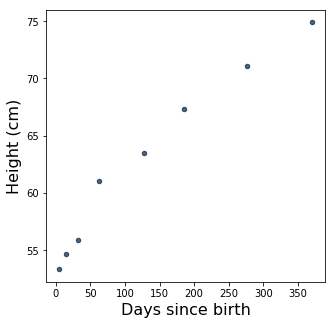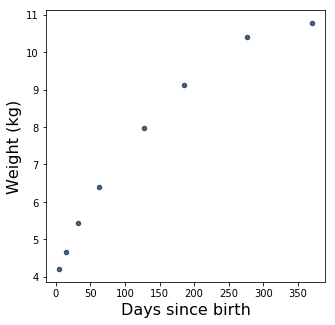How linear are these relationships? We can find out by computing $r$ for each relationship.

In :
heightweight_with_days_standard = Table().with_columns(
"Date", heightweight_with_days.column('Date'),
"Height (standard units)", standard_units(heightweight_with_days.column('Height (cm)')),
"Weight (standard units)", standard_units(heightweight_with_days.column('Weight (kg)')),
"Days since birth (standard units)", standard_units(heightweight_with_days.column('Days since birth'))
)
heightweight_with_days_product = heightweight_with_days_standard.with_columns(
"Product of standardized height and days since birth",
heightweight_with_days_standard.column('Height (standard units)') * heightweight_with_days_standard.column('Days since birth (standard units)'),
"Product of standardized weight and days since birth",
heightweight_with_days_standard.column('Weight (standard units)') * heightweight_with_days_standard.column('Days since birth (standard units)'))
heightweight_with_days_product

Out:
Date Height (standard units) Weight (standard units) Days since birth (standard units) Product of standardized height and days since birth Product of standardized weight and days since birth
2017-07-28 -1.26135 -1.3158 -1.03738 1.3085 1.36498
2017-08-07 -1.08691 -1.13054 -0.957736 1.04097 1.08276
2017-08-25 -0.912464 -0.808628 -0.814374 0.743087 0.658526
2017-09-25 -0.228116 -0.399485 -0.567474 0.12945 0.226697
2017-11-28 0.107349 0.254728 -0.0577429 -0.00619863 -0.0147087
2018-01-26 0.617255 0.728253 0.412165 0.254411 0.30016
2018-04-27 1.12716 1.2537 1.13694 1.28151 1.42538
2018-07-30 1.63707 1.41777 1.88561 3.08686 2.67336
In :
r_days_height = np.mean(heightweight_with_days_product.column('Product of standardized height and days since birth'))
print("r_days_height:", r_days_height)
r_days_weight = np.mean(heightweight_with_days_product.column('Product of standardized weight and days since birth'))
print("r_days_weight:", r_days_weight)

r_days_height: 0.9798241135426988
r_days_weight: 0.9646449280709019


Both of these values for $r$ are pretty close to 1, although a little less so than the relationship between height and weight. Let's plot the regression lines:

In :
heightweight_with_days_standard.scatter("Days since birth (standard units)", "Height (standard units)", label="data")
x = np.array(range(-2, 3))
y = r_days_height * x # <-- the regression equation
plots.plot(x, y, label="regression line", linewidth=1.5)
y = x # <-- linear correlation
plots.plot(x, y, label="linear correlation", linewidth=1.5)
plots.legend()

heightweight_with_days_standard.scatter("Days since birth (standard units)", "Weight (standard units)", label="data")
x = np.array(range(-2, 3))
y = r_days_weight * x # <-- the regression equation
plots.plot(x, y, label="regression line", linewidth=1.5)
y = x # <-- linear correlation
plots.plot(x, y, label="linear correlation", linewidth=1.5)
plots.legend();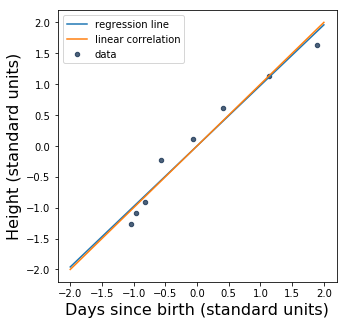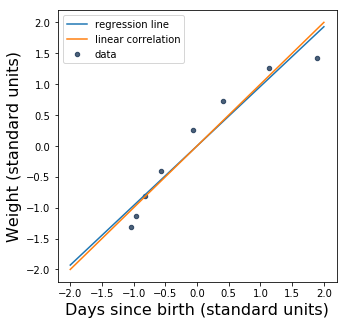Although $r$ is pretty close to 1 in each case, the shapes of the scatter plots look to me like the data would be better modeled by a curved line, especially the second one. Maybe the next part of the course will talk about those.